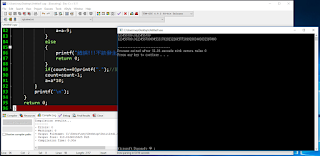## 2016年9月2日 星期五

### 給新手的C++教學 (上冊) - 13 - 3. 進階型別的介紹

1. 浮點數
• 特色：支援小數、有微小誤差、數字範圍大
• 溢位會怎樣：超過最大值會變成inf，超過最小值會變成-inf
• 除以0會怎樣：正數除以0會變成inf、負數除以0會變成-inf，0除以0會變成nan (「not a number」的縮寫)
• 型別有哪些：  名稱 float double long double __float128 占用記憶體 32位元 64位元 96位元 128位元 誤差和數字範圍 占用記憶體越大，誤差越小、數字範圍越大 輸入(scanf) %f %lf 不支援 不支援 輸出(printf) %f %f 不支援 不支援
斜體標示 (針對__float128)：如果要使用此型別，請先參考前一頁並設定好編譯參數，以使用C++11的功能
2. 整數
• 特色：不支援小數、沒有誤差、數字範圍小
• 溢位會怎樣：超過最大值會變成最小值，超過最小值會變成最大值
• 除以0會怎樣：會造成不可預知的錯誤，通常是程式出錯並停止運作
• 型別有哪些：  名稱 bool char short int long long __int128 占用記憶體 8位元 8位元 16位元 32位元 64位元 128位元 數字範圍 $0\sim 1$ $(-2^{占用記憶體-1})\sim (2^{占用記憶體-1}-1)$ 輸入(scanf) 不支援 %c 不支援 %d %lld 不支援 輸入(scanf) 不支援 %c 不支援 %d %lld 不支援
粗體標示 (針對char)：輸入輸出皆以字元的方式進行 (而不是數字)
斜體標示 (針對__int128)：如果要使用此型別，請先參考前一頁並設定好編譯參數，以使用C++11的功能
• 另外，整數還可以用「unsigned」修飾而有更多玩法！意者請Google搜尋「C++ unsigned 用法」

#include<cstdio>
int main()
{
double a,b;
long double c,d,e;
scanf("%lf%lf",&a,&b);
c=a;
d=b;
e=c+d;
double f=(double)e;//請注意，「f」的誤差是「double」等級 (而不是「long double」等級)
printf("%f\n",f);
return 0;
}

#include<cstdio>
bool IsDigit(char c)
{
return '0'<=c&&c<='9';
}
int main()
{
long double a=0;
{
char c;
while(1)
{
scanf("%c",&c);
if(!IsDigit(c))break;
a=a*10+(c-'0');
}
if(c=='.')//如果有小數點
{
long double digit=0.1;
while(c)
{
scanf("%c",&c);
if(!IsDigit(c))break;
a=a+digit*(c-'0');
digit=digit/10;
}
}
}
{
int count=0;
while(a/10>=1)
{
a=a/10;
count=count+1;
}
while(count>=-50)//假設要輸出小數點以下50位數
{
if(0<=a&&a<1)printf("0");
else if(1<=a&&a<2)
{
printf("1");
a=a-1;
}
else if(2<=a&&a<3)
{
printf("2");
a=a-2;
}
else if(3<=a&&a<4)
{
printf("3");
a=a-3;
}
else if(4<=a&&a<5)
{
printf("4");
a=a-4;
}
else if(5<=a&&a<6)
{
printf("5");
a=a-5;
}
else if(6<=a&&a<7)
{
printf("6");
a=a-6;
}
else if(7<=a&&a<8)
{
printf("7");
a=a-7;
}
else if(8<=a&&a<9)
{
printf("8");
a=a-8;
}
else if(9<=a&&a<10)
{
printf("9");
a=a-9;
}
else
{
printf("錯誤!!!不該發生這種情況的!!!\n");
return 0;
}
if(count==0)printf(".");//剛剛輸出的是個位數字，是時候接小數點了
count=count-1;
a=a*10;
}
printf("\n");
}
return 0;
}「double」版本「long double」版本

(版權所有 All copyright reserved)